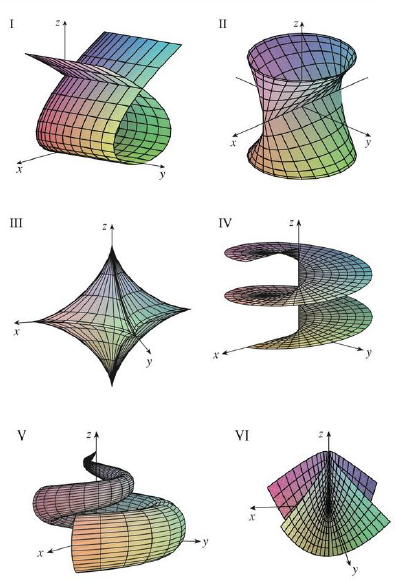Chapter 16.6, Problem 16E

Chapter
Section
Textbook Problem

Match the equations with the graphs labeled I–VI and give reasons for your answers. Determine which families of grid curves have u constant and which have v constant.16. x = (1 − u)(3 + cos v) cos 4πu, y = (1 − u)(3 + cos v) sin 4πu, z = 3u + (1 − u) sin vTo determine

To match: The parametric equations x=(1u)(3+cosv)cos4πu,y=(1u)(3+cosv)sin4πu,z=3u+(1u)sinv with the graphs labeled I-VI in the textbook and to explain the reason for the combination.

Explanation

Given data:

Write the given parametric equations as follows.

x=(1u)(3+cosv)cos4πu,y=(1u)(3+cosv)sin4πu,z=3u+(1u)sinv

The graphs are labeled in the textbook from I to VI.

Determination of grid curves when u is constant:

Consider the parameter u=0 .

Substitute 0 for u in the parametric equations as follows.

x=(10)(3+cosv)cos4π(0),y=(10)(3+cosv)sin4π(0),z=3(0)+(10)sinvx=(3+cosv)(1),y=(3+cosv)(0),z=0+sinvx=3+cosv,y=0,z=sinv

From the expression x=3+cosv,y=0,z=sinv , it is clear that the equation represents the circle with radius 1 in the xz-plane centered at the point (3,0,0) .

Consider the parameter u=12 .

Substitute 12 for u in the parametric equations,

x=(112)(3+cosv)cos4π(12),y=(112)(3+cosv)sin4π(12),z=3(12)+(112)sinvx=(12)(3+cosv)(1),

Still sussing out bartleby?

Check out a sample textbook solution.

See a sample solution

The Solution to Your Study Problems

Bartleby provides explanations to thousands of textbook problems written by our experts, many with advanced degrees!

Get Started

A sample with a mean of M = 8 has X = 56. How many scores are in the sample?

Essentials of Statistics for The Behavioral Sciences (MindTap Course List)

The arc length of y = 3x + 2 from x = 1 to x = 5 is a) 210 b) 52 c) 102 d) 410

Study Guide for Stewart's Single Variable Calculus: Early Transcendentals, 8th

True or False: is a convergent series.

Study Guide for Stewart's Multivariable Calculus, 8th

Explain the distinction between a hypothesis and a prediction.

Research Methods for the Behavioral Sciences (MindTap Course List)

Find each value. log1/28

College Algebra (MindTap Course List)